# Introduction on Synchronous Machines Notes | Study Electrical Machines - Electrical Engineering (EE)

## Electrical Engineering (EE): Introduction on Synchronous Machines Notes | Study Electrical Machines - Electrical Engineering (EE)

The document Introduction on Synchronous Machines Notes | Study Electrical Machines - Electrical Engineering (EE) is a part of the Electrical Engineering (EE) Course Electrical Machines.
All you need of Electrical Engineering (EE) at this link: Electrical Engineering (EE)

Introduction

With the development of the technology and the way in which human labour is getting minimized and the comforts increasing tremendously the use of electrical energy is ever increasing. Basically electric power is the main source of energy for carrying out many functions, as it is a clean and eﬃcient energy source, which can be easily transmitted over long distances. With the availability of Transformer for changing the voltage levels to a very high value (of say 132kV to 400kV) the use of AC power has increased rapidly and the DC power is used only at remote places where AC power cannot be supplied through power lines or cables or for a few social purposes.

A synchronous generator is an electrical machine producing alternating emf (Electromotive force or voltage) of constant frequency. In our country the standard commercial frequency of AC supply is 50 Hz. In U.S.A. and a few other countries the frequency is 60 Hz. The AC voltages generated may be single phase or 3-phase depending on the power supplied. For low power applications single phase generators are preferable. The basic principles involved in the production of emf and the constructional details of the generators are discussed below.

Generation of emf

In 1831 Faraday discovered that an emf can be induced (or generated) due to relative motion between a magnetic ﬁeld and a conductor of electricity. This voltage was termed as the induced emf since the emf is produced only due to motion between the conductor and the magnetic ﬁeld without actual physical contact between them. The principle of electromagnetic induction is best understood by referring to Fig. 1. The magnetic ﬁeld is produced by the two ﬁxed poles one being the north pole from which the magnetic ﬂux lines emerge and enter into the other pole known as the south pole. It was found that the magnitude of the voltage induced in the conductor is proportional to the rate of change of ﬂux lines linking the conductor.
Mathematically it is given as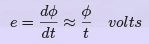(1)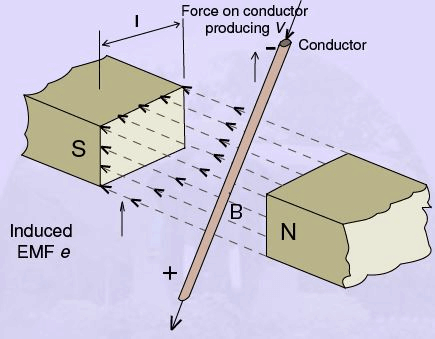Figure 1: Conductor of length ‘l ’ moving through a magnetic ﬁeld B generate an EMF

where
φ = ﬂux in Webers
t = time in seconds
e = average induced emf in volts.

The above Eqn. 1 holds good only when the magnetic circuit is physically the same at the end as at the beginning and also during the period of change of ﬂux linkages as well. In practical rotating machinery, however the change of ﬂux linking each individual conductor during rotation (of either the conductors or the poles) is not clearly deﬁned or cannot be easily measured. It is therefore more convenient to express this rate of change of ﬂux in terms of an average ﬂux density (assumed constant) and the relative velocity between this ﬁeld and a single conductor moving through it. For the conductor of active length l moving with a velocity of v in a magnetic ﬁeld of ﬂux density B , as shown in Fig. 1, the instantaneous induced emf is expressed as,

e = B lv V olts (2)

where B= ﬂux density in Tesla (Wb/m2)
l = active conductor length (m)
v = relative linear velocity between the conductor and the ﬁeld (m/s).
This animation would help to understand the concept for a coil rotating in a magnetic ﬁeld.
Thus the instantaneous voltage e and the average value E of the induced emf are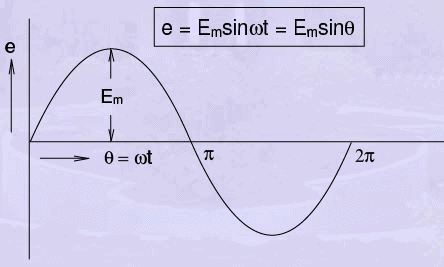Figure 2: Sinusoidal voltage waveform

the same if the ﬂux density B and the relative velocity v are both uniform and constant. In an alternator we want the instantaneous emf to be varying in a sinusoidal manner as shown in Fig. 2. Hence we should have a ﬁeld system which will produce a sinusoidal distribution of ﬂux density in the plane perpendicular to the plane of motion of the conductor.Then,

e = Em sin ωt = Em sin θ                       (3)

We have assumed that the conductor is moved in a direction perpendicular to the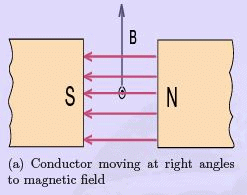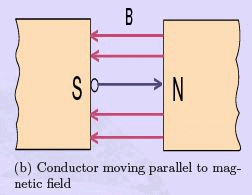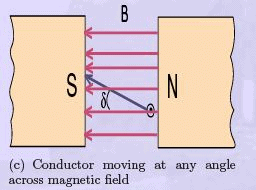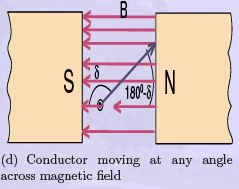Figure 3: Eﬀect of change of ﬂux linkages on induced EMF in a conductor magnetic ﬁeld as shown in Fig. 1. Eqn. 1 or Eqn. 2 are valid only for this mutually orthogonal condition for B and v. The other possible cases of motion of conductor with respect to B are shown in Fig.3 in addition to the mutually orthogonal condition of Fig. 1. When the conductor moves parallel to B, the induced emf will be zero because the rate of change of ﬂux linkage is zero as the conductor does not link any new ﬂux line/lines. To account for this condition of operation, Eqn. 2 must be multiplied by some factor, that takes into account the direction of motion of conductor so as to make ‘e’ zero for this condition of operation although B , l and v are ﬁnite quantities. Intuitively we may infer that this factor must be a sine function as it has a zero value at 0 and also at 180 and a maximum value at 90 .

Indeed the emf equation for the general case of a conductor moving in any direction with respect to the ﬁeld as shown in Fig. 3 is given by
e = B lv sin δ               (4)

where δ is the angle formed between B and v always taking B as the reference. All other quantities are the same as in Eqn. 2.

Direction of induced e.m.f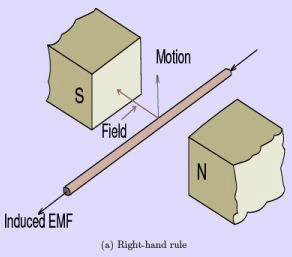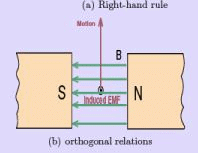Figure 4: Fleming’s right hand rule for direction of induced EMF

The direction of the induced emf is given by Fleming’s Right Hand Rule which states: If the thuMb, First ﬁnger and the seCond ﬁnger of the right hand are stretched out and held in three mutually perpendicular directions such that the First ﬁnger is held pointing in the direction of the magnetic ﬁeld and the thuMb pointing in the direction of motion, then the seCond ﬁnger will be pointing in the direction of the induced emf such that the current ﬂows in that direction. As shown in Fig. 4 the induced emf is in a direction so as to circulate current in the direction shown by the middle ﬁnger. Schematically we indicate the direction of the emf by a dot as shown in Fig. 5(a) to represent an emf so as to send current in a direction perpendicular to the plane of the paper and out of it. A cross will indicate the emf of opposite polarity, see Fig. 5(b). Although the Right Hand Rule assumes the magnetic ﬁled to be stationary, we can also apply this rule to the case of a stationary conductor and moving magnetic ﬁeld, by assuming that the conductor is moving in the opposite direction.
For example, as shown in Fig. 4 the direction of the induced emf will be the same if the poles producing the ﬁeld had been moved upwards.

Electromagnetic Force

The motion of the conductor in a magnetic ﬁeld can be imparted by the application of an external mechanical force to the conductor. In such a case the mechanical work done in moving the conductor is converted to an electric energy in agreement with the law of conservation of energy. The electric energy is not produced by the magnetic ﬁeld since the ﬁeld is not changed or destroyed in the process. The name electro mechanical energy conversion is given to the process of converting energy from mechanical form obtained from a prime mover, such as an IC engine, water/steam turbine etc, into electric energy.

The emf induced in the conductor will circulate a current through it if a closed circuit is formed by an external connection. The direction of the current ﬂowing in the conductor will be such as to oppose the cause of it as stated by Lenz’s Law. A current carrying conductor located in a magnetic ﬁeld will experience a force given by Biot-savart’s law:

f = Bli                 (5)

In other words, whenever a change in ﬂux linkages occur, an emf is induced that tends to set up a current in such a direction as to produce a magnetic ﬂux that opposes the cause of it. Thus if a current carrying conductor is placed in a magnetic ﬁeld as shown in Fig. 5 the current tends to produce a magnetic ﬁeld in the direction shown by the dotted circles.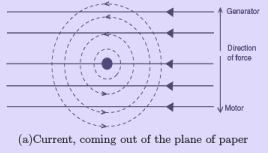(a)Current, coming out of the plane of paper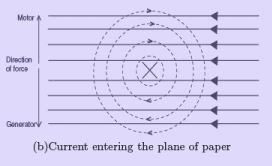(b)Current entering the plane of paper

Figure 5: Force on a current carrying conductor in a magnetic ﬁeld

The direction of the ﬂux lines around the current carrying conductor can be easily determined by Corkscrew Rule - which states that the ﬂux lines will be in the same direction as the rotation of a right threaded screw so as to advance in the direction of ﬂow of current.
As a result the magnetic ﬁeld, for the case shown in Fig. 5(a), is strengthened at the top and weakened at the bottom of the conductor, thereby setting up a force to move the conductor downwards. For the case of a Generator, the conductor must be moved up against this counter force or the opposing force. Similarly the current is to be supplied to the conductor against the emf generated (known as the counter emf or back emf ) in the conductor as it moves due to the motor action. Thus, the same machine can be operated as a generator or a motor, depending on whether we supply mechanical power or electrical power to it, respectively.

Elementary AC. Generators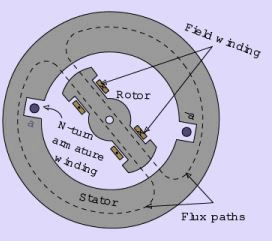Figure 6: Elementary synchronous generator

The generators shown in Fig. 1 and Fig. 4 and discussed in the earlier sections are clearly impractical for a number of reasons. The main reason is that such generators require a prime mover that imparts linear or reciprocating motion to the conductor. Most of the commercial prime movers provide rotary motion in the commercial generators. The conductors of most commercial generators are rotated about a central axis of a shaft. The conductors are housed in slots cut in a cylindrical structure (made of magnetic material) known as the armature.

The armature is supported at both ends by means of bearings attached to the shaft that goes through the center of the armature. The armature is rotated inside the ﬁeld structure by providing a small gap between these two members. This gap is known as the air gap and is usually of the order of 1 to 1.5 cms. If the air gap is maintained constant throughout the spread of the pole arc, we have a fairly constant ﬂux density under it in a plane perpendicular to the plane of the conductor’s motion. i.e. in a radial direction with respect to the ﬁeld and armature structure. Since the emf is also proportional to B , the ﬂux density in the air gap of AC generators is arranged to be distributed as closely to a sine wave as possible by suitable shaping (chamfering as it is technically known) of the pole shoe. Since the relative motion between the conductors and the magnetic ﬂux lines is responsible for the production of emf, it is immaterial whether the conductors are rotated or the magnetic ﬂux producing poles are rotated. In most of the alternators it is the ﬁeld that is rotated rather than the conductors. In an alternator the external connection to the load can be taken directly from the conductors since there is no need for any rectiﬁcation as in a DC generator. In a DC generator the rectiﬁcation of the emf is achieved through a mechanical rectiﬁer—- the commutator and brush arrangement. Moreover the load current supplied by the alternator can be easily supplied from stationary coils without any diﬃculty as there will be no sparking and wear and tear of the brushes and slip rings. Where as the low values of D.C excitation current to the ﬁeld coils can be easily sent through the slip rings and brush arrangement. Thus the usual arrangement in an elementary synchronous generator is as shown in Fig. 6. The conductors are housed in slots cut in the armature structure. Only a single coil of N turns, indicated in its cross-section by the two coil sides a and -a placed in diametrically opposite slots on the inner periphery of the stator (i.e. the armature, as it is a stationary member here) is shown in Fig. 6.

The conductors forming these coil sides are parallel to the shaft of the machine and are connected in series by end connections (not shown in the ﬁgure ). The coils are actually formed by taking a continuous copper wire of suitable cross section round a diamond shaped bobbin. The completed coil is shown in Fig. 7. The copper wire is usually of ﬁne linen covered, cotton covered or enamel covered so as to have suﬃcient insulation between the conductors of the same coil. The actual layout and interconnection of various coils so as to obtain the required voltage from the synchronous machine (alternator) is presented in the following section.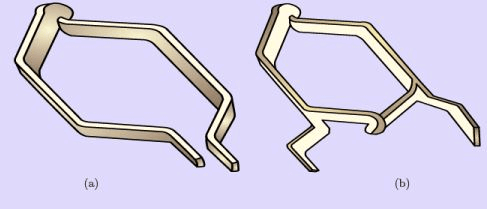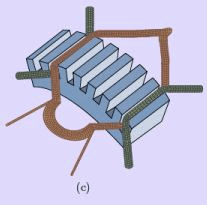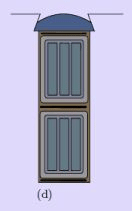Figure 7: The completed coil

The document Introduction on Synchronous Machines Notes | Study Electrical Machines - Electrical Engineering (EE) is a part of the Electrical Engineering (EE) Course Electrical Machines.
All you need of Electrical Engineering (EE) at this link: Electrical Engineering (EE)Use Code STAYHOME200 and get INR 200 additional OFF

## Electrical Machines

62 docs|20 tests

Track your progress, build streaks, highlight & save important lessons and more!

,

,

,

,

,

,

,

,

,

,

,

,

,

,

,

,

,

,

,

,

,

;